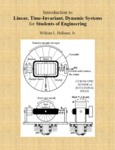## Open Textbooks#### Title

Introduction to Linear/ Time-Invariant/ Dynamic Systems for Students of Engineering

#### Contributors

William Hallauer - Author

#### Description

This is a complete college textbook/ including a detailed Table of Contents/ seventeen Chapters (each with a set of relevant homework problems)/ a list of References/ two Appendices/ and a detailed Index. The book is intended to enable students to: Solve first-/ second-/ and higher-order/ linear/ time-invariant (LTI) or­dinary differential equations (ODEs) with initial conditions and excitation/ using both time-domain and Laplace-transform methods; Solve for the frequency response of an LTI system to periodic sinusoi­dal excitation and plot this response in standard form; Explain the role of the time constant in the response of a first-order LTI system/ and the roles of natural frequency/ damping ratio/ and resonance in the response of a second-order LTI system; Derive and analyze mathematical models (ODEs) of low-order me­chanical systems/ both translational and rotational/ that are com­posed of inertial elements/ spring elements/ and damping devices; Derive and analyze mathematical models (ODEs) of low-order electri­cal circuits composed of resistors/ capacitors/ inductors/ and op­erational amplifiers; Derive (from ODEs) and manipulate Laplace transfer functions and block diagrams representing output-to-input relationships of discrete ele­ments and of systems; Define and evaluate stability for an LTI system; Explain proportional/ integral/ and derivative types of feedback control for single-input/ single-output (SISO)/ LTI systems; Sketch the locus of characteristic values/ as a control parameter varies/ for a feedback-controlled SISO/ LTI system; Use MATLAB as a tool to study the time and frequency responses of LTI systems. The book's gen­eral organization is: Chapters 1-10 deal primarily with the ODEs and behaviors of first-order and second-order dynamic systems; Chapters 11 and 12 discuss the ODEs and behaviors of mechanical systems having two degrees of freedom/ i.e./ fourth-order systems; Chapters 13 and 14 introduce classical feedback control; Chapter 15 presents the basic features of proportional/ in­tegral/ and derivative types of classical control; Chapters 16 and 17 discuss methods for analyzing the stability of classical control systems. The general minimum prerequisite for understanding this book is the intellectual matur­ity of a junior-level (third-year) college student in an accredited four-year engineering curriculum. A mathematical second-order system is represented in this book primarily by a single second-order ODE/ not in the state-space form by a pair of coupled first-order ODEs. Similarly/ a two-degrees-of-freedom (fourth-order) system is represented by two coupled second-order ODEs/ not in the state-space form by four coupled first-order ODEs. The book does not use bond graph modeling/ the general and powerful/ but complicated/ modern tool for analysis of complex/ multidisciplinary dynamic systems. The homework problems at the ends of chapters are very important to the learning objectives/ so the author attempted to compose problems of practical interest and to make the problem statements as clear/ correct/ and unambiguous as possible. A major focus of the book is computer calculation of system characteristics and responses and graphical display of results/ with use of basic (not advanced) MATLAB commands and programs. The book includes many examples and homework problems relevant to aerospace engineering/ among which are rolling dynamics of flight vehicles/ spacecraft actuators/ aerospace motion sensors/ and aeroelasticity. There are also several examples and homework problems illustrating and validating theory by using measured data to identify first- and second-order system dynamic characteristics based on mathematical models (e.g./ time constants and natural frequencies)/ and system basic properties (e.g./ mass/ stiffness/ and damping). Applications of real and simulated experimental data appear in many homework problems. The book contains somewhat more material than can be covered during a single standard college semester/ so an instructor who wishes to use this as a one-semester course textbook should not attempt to cover the entire book/ but instead should cover only those parts that are most relevant to the course objectives.

#### Subject 1

Engineering - Electrical Engineering

#### Subject 2

Engineering - Electrical Engineering

#### Publisher

A.T. Still University

#### Resources

Open Textbook Library